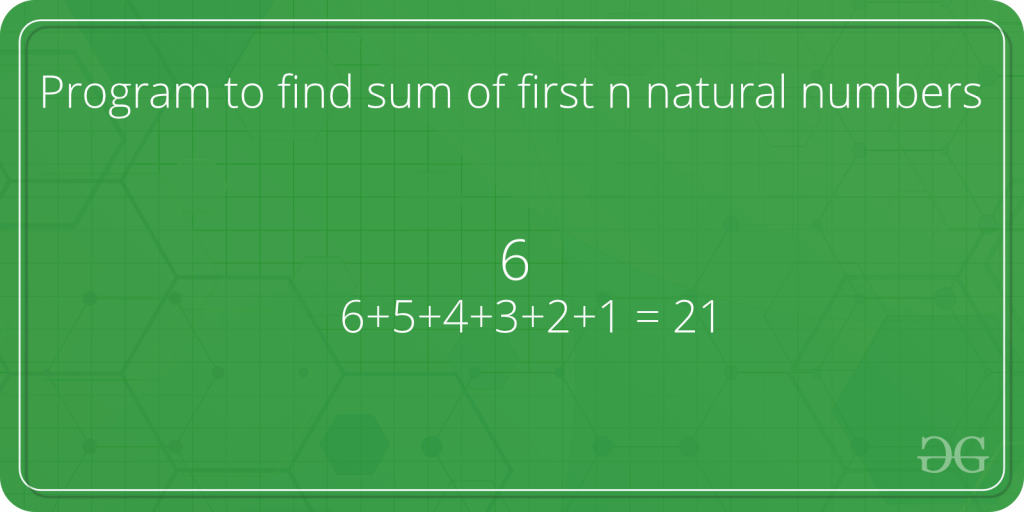# Sum of natural numbers using recursionGiven a number n, find sum of first n natural numbers. To calculate the sum, we will use a recursive function recur_sum().

Examples :

```Input : 3
Output : 6
Explanation : 1 + 2 + 3 = 6

Input : 5
Output : 15
Explanation : 1 + 2 + 3 + 4 + 5 = 15
```

## Recommended: Please try your approach on {IDE} first, before moving on to the solution.

Below is code to find the sum of natural numbers up to n using recursion :

## C++

 `// C++ program to find the  ` `// sum of natural numbers up ` `// to n using recursion ` `#include ` `using` `namespace` `std; ` ` `  `// Returns sum of first  ` `// n natural numbers ` `int` `recurSum(``int` `n) ` `{ ` `    ``if` `(n <= 1) ` `        ``return` `n; ` `    ``return` `n + recurSum(n - 1); ` `} ` ` `  `// Driver code ` `int` `main() ` `{ ` `    ``int` `n = 5; ` `    ``cout << recurSum(n); ` `    ``return` `0; ` `} `

## Java

 `// Java program to find the  ` `// sum of natural numbers up ` `// to n using recursion ` `import` `java.util.*; ` `import` `java.lang.*; ` ` `  `class` `GFG ` `{ ` ` `  `    ``// Returns sum of first  ` `    ``// n natural numbers ` `    ``public` `static` `int` `recurSum(``int` `n) ` `    ``{ ` `        ``if` `(n <= ``1``) ` `            ``return` `n; ` `        ``return` `n + recurSum(n - ``1``); ` `    ``} ` `     `  `    ``// Driver code ` `    ``public` `static` `void` `main(String args[]) ` `    ``{ ` `        ``int` `n = ``5``; ` `        ``System.out.println(recurSum(n)); ` `    ``} ` `} ` ` `  `// This code is contributed by Sachin Bisht `

## Python

 `# Python code to find sum  ` `# of natural numbers upto ` `# n using recursion ` ` `  `# Returns sum of first ` `# n natural numbers ` `def` `recurSum(n): ` `    ``if` `n <``=` `1``: ` `        ``return` `n ` `    ``return` `n ``+` `recurSum(n ``-` `1``) ` ` `  `# Driver code ` `n ``=` `5` `print``(recurSum(n)) `

## C#

 `// C# program to find the  ` `// sum of natural numbers ` `// up to n using recursion ` `using` `System; ` ` `  `class` `GFG ` `{ ` ` `  `    ``// Returns sum of first  ` `    ``// n natural numbers ` `    ``public` `static` `int` `recurSum(``int` `n) ` `    ``{ ` `        ``if` `(n <= 1) ` `            ``return` `n; ` `        ``return` `n + recurSum(n - 1); ` `    ``} ` `     `  `    ``// Driver code ` `    ``public` `static` `void` `Main() ` `    ``{ ` `        ``int` `n = 5; ` `        ``Console.WriteLine(recurSum(n)); ` `    ``} ` `} ` ` `  `// This code is contributed by vt_m `

## PHP

 ` `

Output :

`15 `

Attention reader! Don’t stop learning now. Get hold of all the important DSA concepts with the DSA Self Paced Course at a student-friendly price and become industry ready.

My Personal Notes arrow_drop_upIf you like GeeksforGeeks and would like to contribute, you can also write an article using contribute.geeksforgeeks.org or mail your article to contribute@geeksforgeeks.org. See your article appearing on the GeeksforGeeks main page and help other Geeks.

Please Improve this article if you find anything incorrect by clicking on the "Improve Article" button below.

Improved By : jit_t

Article Tags :
Practice Tags :

1

Please write to us at contribute@geeksforgeeks.org to report any issue with the above content.